## 6 Example solution

06.613GPPSubstitution and muting of lost frames for encanced full rate speech traffic channelsTS

The C-code of the following example is embedded in the bit exact software of the enhanced full rate codec.

## 6.1 Example solution for substitution and muting of lost speech frames

This example solution for substitution and muting is based on a state machine with seven states (figure 1).

The system starts in state 0. Each time a bad frame is detected, the state counter is incremented by one and is saturated when it reaches 6. Each time a good speech frame is detected, the state counter is reset to zero, except when we are in state 6, where we set the state counter to 5. The state indicates the quality of the channel: the bigger the state counter, the worse the channel quality is. The control flow of the state machine can be described with the following C-code (BFI = bad frame indicator, State = state variable):

if(BFI != 0 )

State = State + 1;

else if(State == 6)

State = 5;

else

State = 0;

if(State > 6 )

State = 6;

In addition to this state machine, the Bad Frame Flag from the previous frame is checked (PrevBFI). The processing depends on the value of the State-variable. In states 0 and 5, the processing depends also on the two flags BFI and PrevBFI.

The procedure can be described as follows: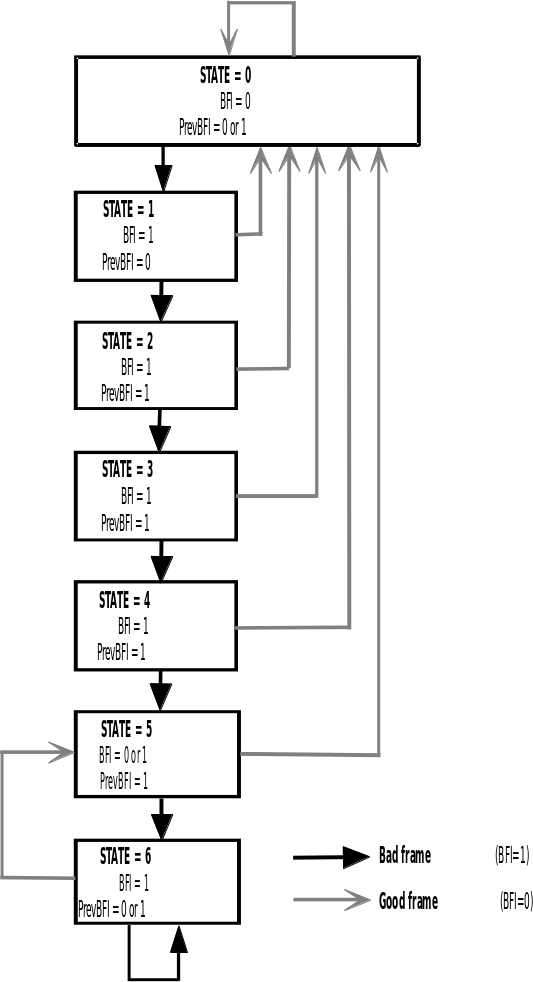Figure 1: State machine for controlling the bad frame substitution

BFI = 0, PrevBFI = 0, State = 0

No error is detected in the received or in the previous received speech frame. The received speech parameters are used normally in the speech synthesis. The current frame of speech parameters is saved.

BFI = 0, PrevBFI = 1, State = 0 or 5

No error is detected in the received speech frame but the previous received speech frame was bad. The LTP-gain and fixed codebook gain are limited below the values used for the last received good subframe:

(1)

where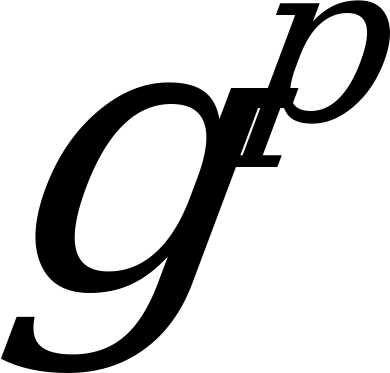= current decoded LTP-gain,= LTP-gain used for the last good subframe (BFI = 0), and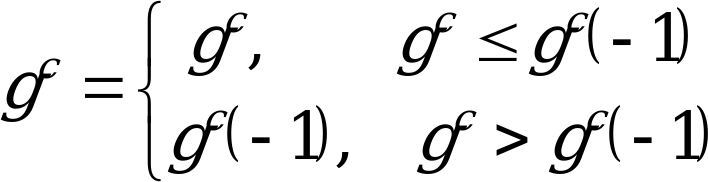(2)

where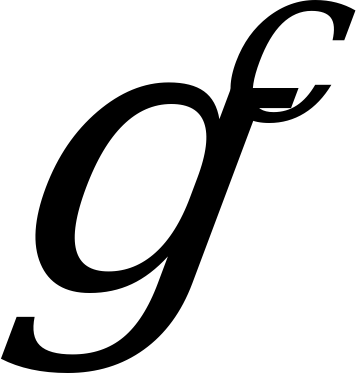= current decoded fixed codebook-gain and = fixed codebook gain used for the last good subframe (BFI = 0).

The rest of the received speech parameters are used normally in the speech synthesis. The current frame of speech parameters is saved.

BFI = 1, PrevBFI = 0 or 1, State = 1…6

An error is detected in the received speech frame and the substitution and muting procedure is started. The LTP-gain and fixed codebook gain are replaced by attenuated values from the previous subframes:

(3)

where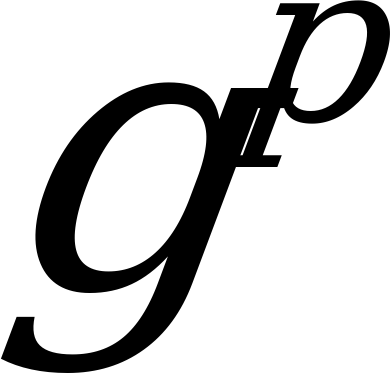= current decoded LTP-gain,= LTP-gains used for the last n subframes, median5() = 5-point median operation, P(state) = attenuation factor (P(1) = 0.98, P(2) = 0.98, P(3) = 0.8, P(4) = 0.3, P(5) = 0.2, P(6) = 0.2), state = state number, and

(4)

where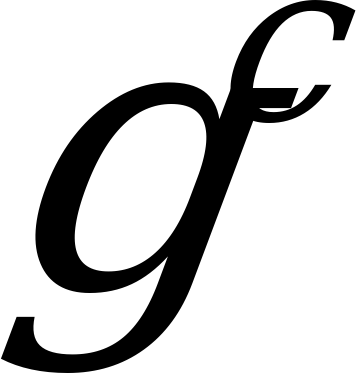= current decoded fixed codebook gain,= fixed codebook gains used for the last n subframes, median5() = 5-point median operation, C(state) = attenuation factor (C(1) = 0.98, C(2) = 0.98, C(3) = 0.98, C(4) = 0.98, C(5) = 0.98, C(6) = 0.7), and state = state number.

The higher the state value is, the more the gains are attenuated. Also the memory of the predictive fixed codebook gain is updated by using the average value of the past four values in the memory:

(5)

The past LSFs are used by shifting their values towards their mean:

(6)

where a = 0.95, lsf_q1 and lsf_q2 are two sets of LSF-vectors for current frame, past_lsf_q is lsf_q2 from the previous frame, and mean_lsf is the average LSF-vector.

The LTP-lag values are replaced by the past value from the 4th subframe of the previous frame.

The received fixed codebook excitation pulses from the erroneous frame are always used as such.

## 6.2 Example solution for substitution and muting of lost SID frames

The first lost SID frame is replaced by the last valid SID frame.

For subsequent lost SID frames, the last valid SID frame is repeated, but the fixed codebook gain is decreased with a constant value of ‑3 dB in each frame down to the minimum value of 0. This value is maintained if additional lost SID frames occur.

Annex A (informative):
Change Request History

 Change history SMG No. TDoc. No. CR. No. Clause affected New version Subject/Comments SMG#22 4.0.1 ETSI Publication SMG#20 5.1.2 Release 1996 version SMG#27 6.0.0 Release 1997 version SMG#29 7.0.0 Release 1998 version 7.0.1 Version update to 7.0.1 for Publication SMG#31 8.0.0 Release 1999 version 8.0.1 Update to Version 8.0.1 for Publication